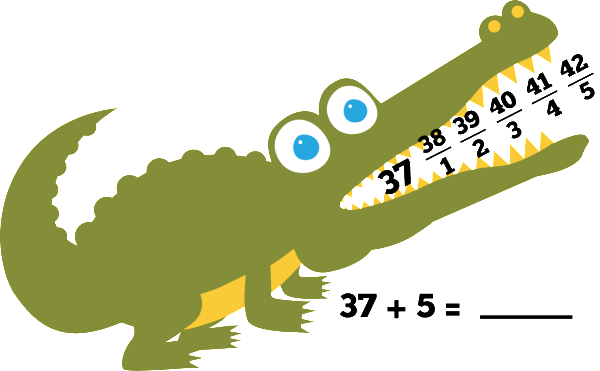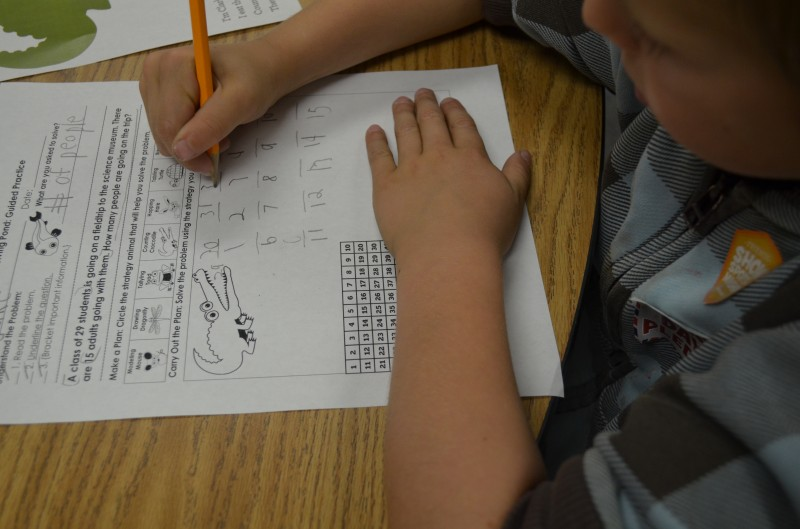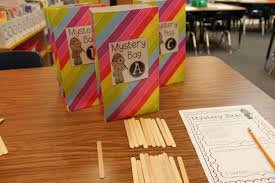# Introducing Clark Counting Crocodile

Mar 3rd 2014Start with the largest number, and draw lines for the remaining amount.
Count on for addition, count back for subtraction;Clark Counting Crocodile is the second strategy animal in our Problem-Solving Pond: A Common Core Math Strategy Unit. The Problem-Solving Pond  was created to help teachers overcome Common Core math challenges and employ problem-solving strategies with confidence and fidelity.  Read more about Clark’s strategy below or download the complete unit here.

WHAT is counting?As students build their number sense and fluency, they are able to solve a story problem with counting.  In this strategy, students will learn to count on for addition and count back for subtraction using a 100’s chart for support as needed.

WHY is counting important?Counting is a crucial strategy because it helps students explore the relationships and patterns between numbers. Students need to recognize the order of numbers so they can understand that it is more efficient to start with bigger number. For example, in order to solve “4+27,” they should start counting from 27 and progress to “28, 29, 30, 31” instead of starting from 4 and progressing to 31, because in the latter case, they would have to count much more, increasing the possibility of errors. Proficient counting lays the foundation for number sense and place value.HOW do I teach counting? Explain that Clark helps mathematicians solve problems by counting on to find the total or counting back to find the difference.

• Counting back for subtraction: Students start with the largest number in the problem; they put that number in Clark’s mouth and then draw the number of lines for the second number in the problem (if the second number is 14, students would draw 14 lines). Students then label the lines (below the line) and count back, writing each number above the line. Students circle the answer and write equation below to solidify understanding.

WHEN should I use counting? This strategy isideal for problems that include smaller numbers.  Most teachers use this strategy with K-2 mathematicians, but this is also beneficial for older students as it works for money, multiplication and division (included in unit).